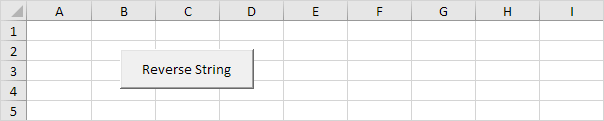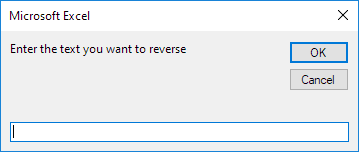# Reverse Strings

Below we will look at a program in Excel VBA that can reverse strings.

Situation:Place a command button on your worksheet and add the following code lines:

1. First, we declare four variables. One variable called text of type String, one variable called reversedText also of type String, one variable called length of type Integer, and one variable called i of type Integer.

Dim text As String, reversedText As String, length As Integer, i As Integer

2. We initialize two variables. We use the InputBox function to get a text string from the user. We use the Len function in Excel VBA to get the length of a string.

text = InputBox("Enter the text you want to reverse")
length = Len(text)3. We start a For Next loop.

For i = 0 To length - 1

4. Now comes the simple trick. We take the last character from text and place it at the front of ReversedText. We can use the Mid function in Excel VBA to extract a character from a string. We use the & operator to concatenate (join) two strings.

reversedText = reversedText & Mid(text, (length - i), 1)

5. Don't forget to close the loop.

Next i

Example: text = "Car". The length of text is 3. For i = 0 to 2, we extract the substring of text starting at position length - i with length 1. Thus, for i = 0, Mid(text, 3, 1) equals r. We place r at the first position of reversedText. For i = 1, Mid(text, 2, 1) equals a. We add a to reversedText which becomes ra. For i  = 2, Mid(text, 1, 1) equals C. We add C to reversedText which becomes raC.

6. Finally, we display reversedText using a MsgBox.

msgbox reversedText

7. Test the program.

Result:Go to Next Chapter: Date and Time